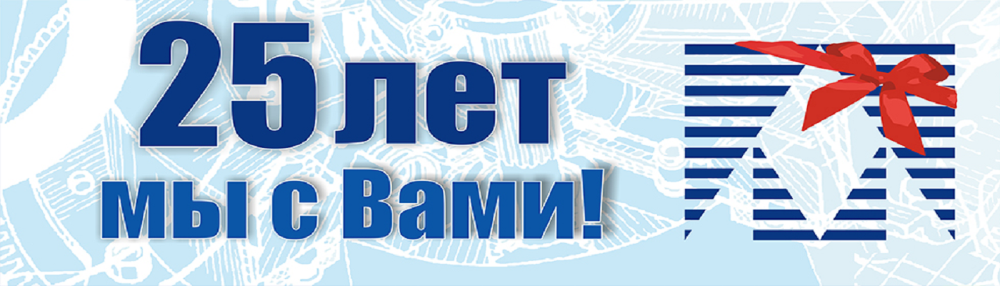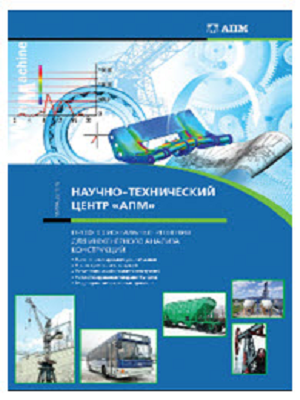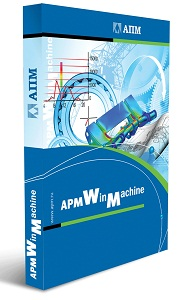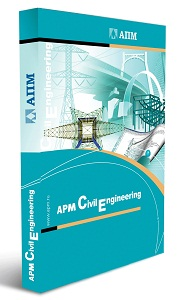PROJECT ENGG » SCIENTIFIC-TECHNICAL CENTER » APM WINMACHINE 16V

# The list of new features and capabilities "line of software products the APM » V16

APM Structure3D

The module for calculating the stress-strain state, stability, proper and forced oscillations of parts and structures by the finite element method

Functional

Topological optimization of structures:

• The new formulation of the problem is the minimization of a mass with stress limitation;

• The possibility to carry out topological optimization for structures consisting of rod CEs is added;

• Additional technological limitations are introduced: "maximum thickness", "symmetry", "stamping";

• Realized the accounting of volumetric (for example, gravity) and surface forces (for example, pressure on the surface of solid-state cells)

Calculations:

• When calculating the eigenfrequencies of preloaded structures, the use of force factors derived from nonlinear calculation is realized;

• Calculation of forced oscillations of structures is possible with allowance for natural frequencies calculated with preloading;

• When testing the load-bearing capacity of steel structural elements, new opportunities have appeared:

• the ultimate flexibility of the element - is calculated automatically;

• in one constructive element it is allowed to place disconnected rods;

• For the sake of clarity of results, color maps of utilization factors

• Calculation of FSI - analysis of VAT taking into account the field of pressures and / or temperatures from APM FGA

• Non-linear calculation: "Cracks - life / death of elements" - added the ability to analyze structures for strength and crack resistance in the construction area (for example, the calculation of reinforced concrete beam)

Stationary thermal analysis:

• Added boundary conditions of the following types: "Flow rate", "Heat flow", "Radiation", "Convection";

• The possibility of choosing the solvers of the SLAE of stationary thermal conductivity

Calculations of electromagnetic fields:

• New solvers of SLAU for magnetostatics and electrostatics are available

Calculations using video cards (CUDA technology):

Implemented the application of CUDA technology for the following types of calculations:

• static calculation;

• calculation of stability;

• calculation of natural frequencies;

• Post-processing results after topological optimization of structures

Calculations of RC structures:

• A verification and design calculation of the cross reinforcement of
RC plates was carried out. Corresponding changes were made in the RC of the CE;

• Calculation of DCS for lateral reinforcement;

• The output of transverse reinforcement of plates on the reinforcement card was added

Work with plate-like cells:

• The command for drawing a rectangular plate with a hole is added;

• The calculation of transverse forces in plates is realized;

• Calculation of shearing shear stresses and deformations for plates (such as MITC)

New objects for constructing computational models:

• Import BDF | DAT grid grid from the command line;

• Import of second-order solid prisms and pyramids from BDF | DAT files

Operation of object selection / editing:

• A new algorithm for "highlighting / separating" the frame;

• "Isolation / dissolution" by an arbitrary circuit;

• "Isolation / dissolution" according to the normal (for plates and TV KE);

• Selection groups;

• Operation "Editing":
- captures objects without their prior selection;
- copies objects when the Shift key is pressed;
- can move an object to a certain distance specified by the
user.

Operations with grid CE: "Integrate a crack in the model (4 nodal tetrahedra)"

• The possibility of calculating the J-integral in static calculations is added;

• The possibility of calculating the number of cycles before Paris destruction in fatigue problems is added (in case the nature of load variation is repeatedly variable);

• The possibility of calculating the reserve factors for random loads in fatigue problems (in case the nature of load variation is random or stochastic) is added;

Interface

The combination of the commands "rod by nodes" and "rod along the length and angle" was carried out. Additionally, it became possible to draw parallel and perpendicular rods to previously selected

The ability to enter material properties for the calculation of fatigue fracture mechanics (constants C and n)

Support for the work of combinations of "Hot keys":

• Selecting the whole construction Ctrl + A

• Canceling the operation Ctrl + Z

• Repeat undo operation Ctrl + Y

In order to increase the ease of use in the "Calculation" dialog, it is possible to "open / hide" the properties of a particular calculation

Added the ability to replace the bottom of the scale on the results map with a white color, which helps visually "highlight" the most stressed places

The output of the upper limit on the safety factor maps is limited to 10APM Studio

Pre- and postprocessor for calculating three-dimensional solid and shell models

The process of generation of the boundary conditions (the last stage in the construction of the grid-grid)

The possibility of carrying out non-linear analysis (geometric nonlinearity, contact interaction)

For the calculations, solvers are built on CUDA technology

Implemented reading of STEP 242 protocol files

Added a special mode for generating a grid grid - "Immersion". The mode is necessary for the automatic "immersion" of the part into the environment and the joint splitting of the part
and the medium into the FE (operation in the APM FGA mode)

APM FGA

Analysis of flows of liquids and gases

The boundary condition "Expenditure" is realized, which is represented in the variants of "volumetric flow" or "mass flow" and can be set on the surface in the form of specific or total values

Implemented a new functional "Movable wall" in the boundary condition "Speed"

The task of "normal / relative conditions"

Embedded drawing of vector results maps on the nodes of the calculation model

The possibility of taking into account earlier obtained results as initial data for the newly conducted Navier-Stokes flow analysis is realized

The automatic transmission of the results of the analysis of the Navier-Stokes flows (pressure and temperature fields) as loads for calculating the stress-strain state for solids (calculation of FSI)

APM ECA

Calculation of electrical circuits

The possibility of creating an electric circuit model using "subsystems" loaded from previously created files is realized. The "subsystem" file must have a link to the main file of the electric circuit model - in this case it can be used in several models at once and any changes in it will affect the operation of all models of electrical circuits. Either the file of the "subsystem" is integrated into the main file of the electric circuit model - the changes in it will only lead
to a local change in the operation of the model of a particular electrical circuit

Each "subsystem" can have a certain set of exported properties

Implemented the ability to specify the properties of elements through calculated mathematical expressions, which can include variables

The integration with the execution environments of high-level programming languages ​​is organized. The current version works with Julia 0.5.

Added the ability to create programmable elements, the function of which is described as a program in a third-party programming language. This type of element can be reused in the current document. Programmatically described element type can be exported and used in other models of electrical circuits

The possibility of using the constructed model of an electrical circuit in an external program is realized. A program in a supported programming language can change the values ​​of the properties of model elements, environment variables and call different types of calculation for the constructed model

A set of methods for solving nonlinear nonsmooth rigid problems includes high-performance methods: Nonexactry Newton (with variations), Trust region

Organized the creation of user libraries of elements ("subsystems") and their storage. It is possible to add the most frequently used "subsystems" to the toolbar in addition to the basic### APM WinMachine

CAD \ CAE computer-aided calculation and design of machine parts, machinery, construction elements and components, engineering facilities and equipment### APM Civil Engineering

CAD \ CAE computer-aided design and analysis of metal, concrete and wooden structures of civil and industrial use

info@paviathintegratedsolution.com www.paviathintegratedsolution.com